# Measures of Central Tendency.ppt

30 de May de 2023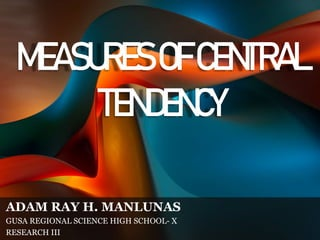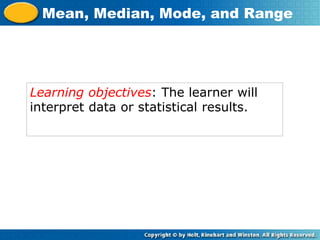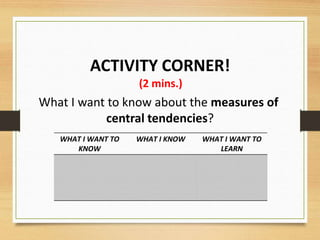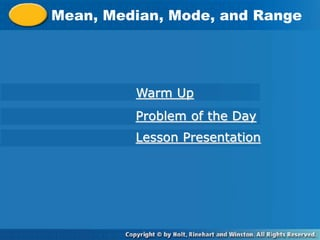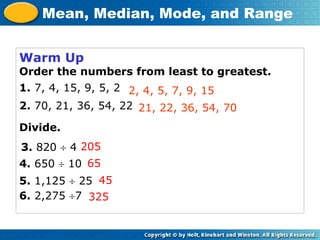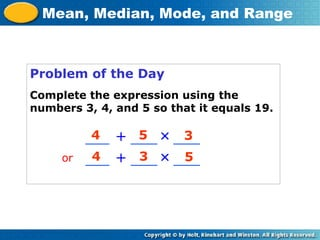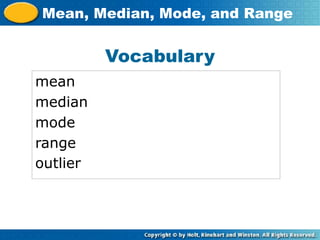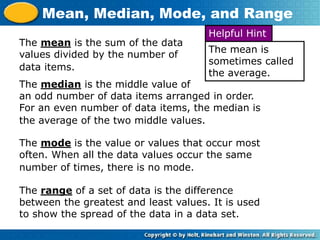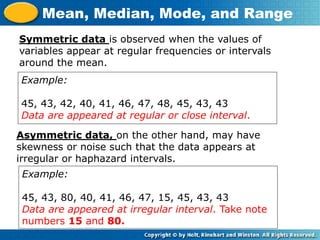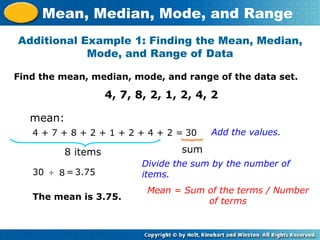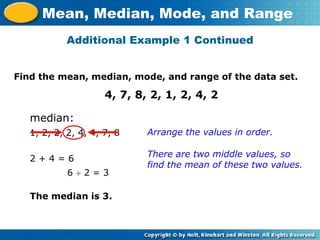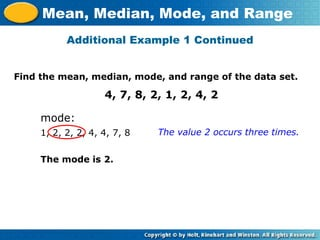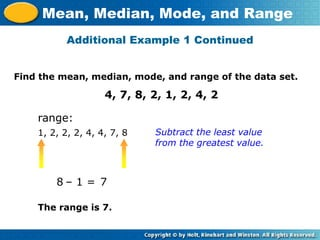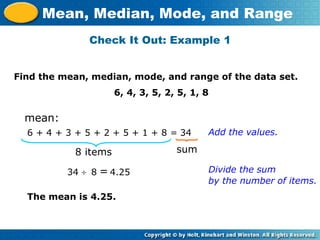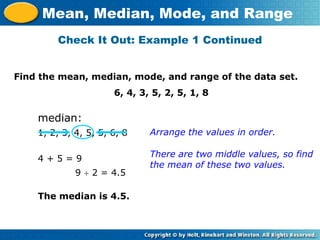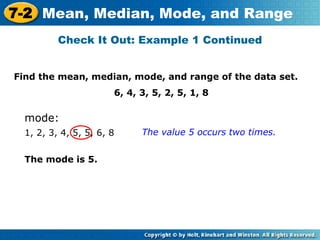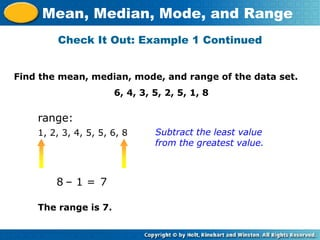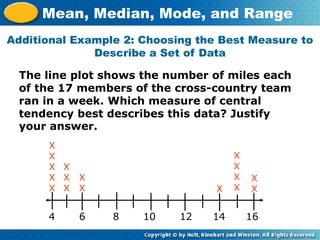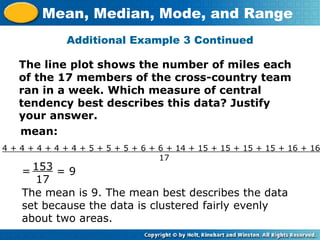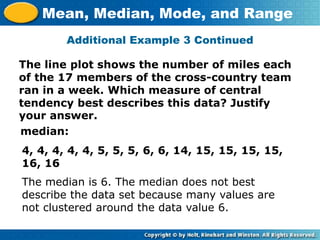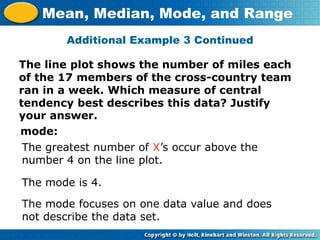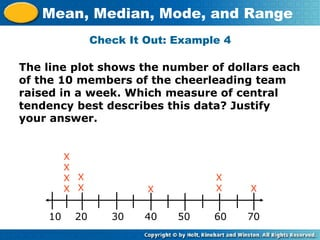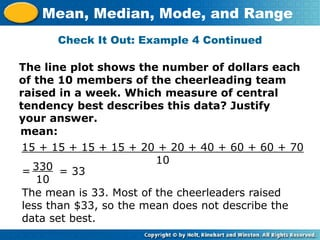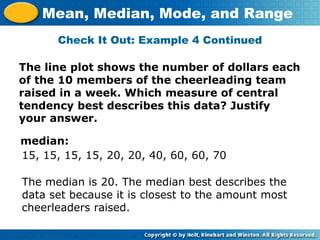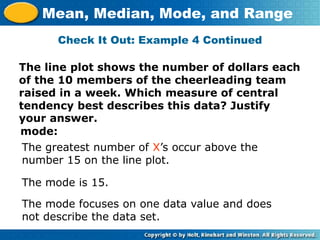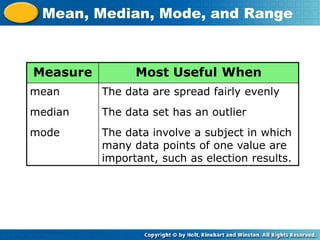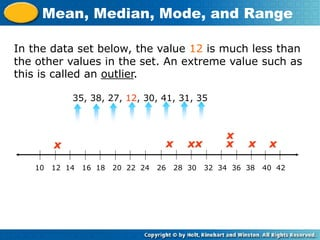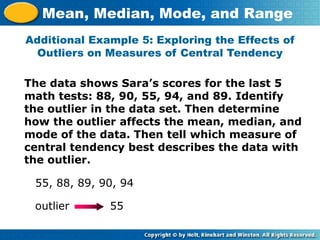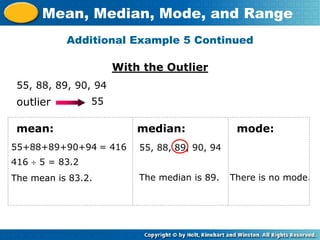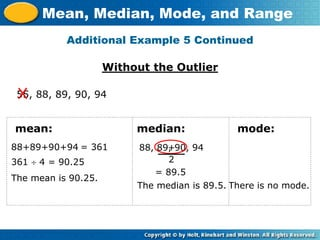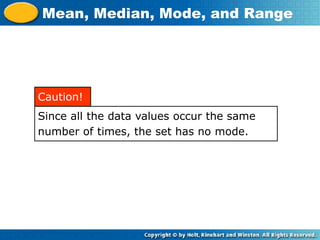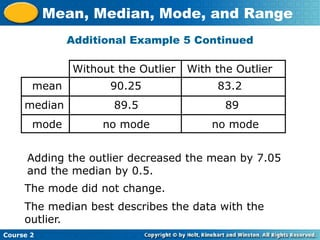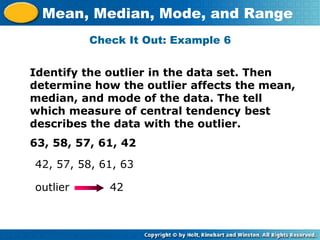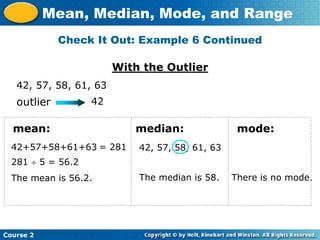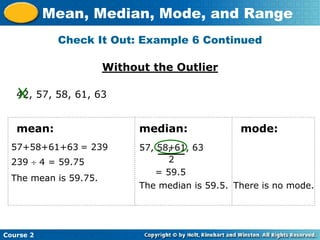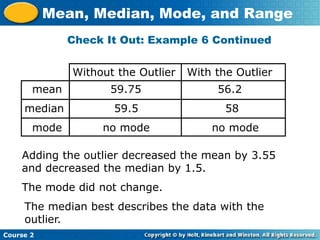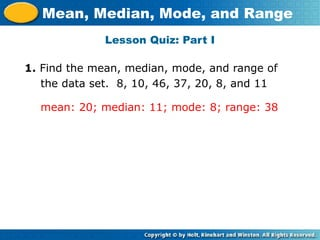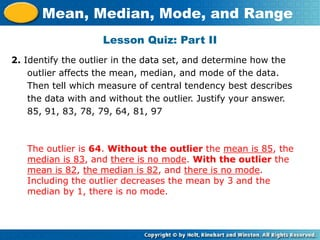1 de 41

### Measures of Central Tendency.ppt

• 1. MEASURESOFCENTRAL TENDENCY ADAM RAY H. MANLUNAS GUSA REGIONAL SCIENCE HIGH SCHOOL- X RESEARCH III
• 2. Learning objectives: The learner will interpret data or statistical results. Mean, Median, Mode, and Range
• 3. ACTIVITY CORNER! (2 mins.) WHAT I WANT TO KNOW WHAT I KNOW WHAT I WANT TO LEARN What I want to know about the measures of central tendencies?
• 4. Mean, Median, Mode, and Range Warm Up Problem of the Day Lesson Presentation
• 5. Warm Up Order the numbers from least to greatest. 1. 7, 4, 15, 9, 5, 2 2. 70, 21, 36, 54, 22 Divide. 2, 4, 5, 7, 9, 15 21, 22, 36, 54, 70 205 65 Mean, Median, Mode, and Range 45 325 6. 2,275 7 5. 1,125  25 4. 650  10 3. 820  4
• 6. Problem of the Day Complete the expression using the numbers 3, 4, and 5 so that it equals 19. +  4 5 3 Mean, Median, Mode, and Range 4 3 5 +  or
• 8. The mean is the sum of the data values divided by the number of data items. The median is the middle value of an odd number of data items arranged in order. For an even number of data items, the median is the average of the two middle values. The mode is the value or values that occur most often. When all the data values occur the same number of times, there is no mode. The range of a set of data is the difference between the greatest and least values. It is used to show the spread of the data in a data set. Mean, Median, Mode, and Range The mean is sometimes called the average. Helpful Hint
• 9. Symmetric data is observed when the values of variables appear at regular frequencies or intervals around the mean. Example: 45, 43, 42, 40, 41, 46, 47, 48, 45, 43, 43 Data are appeared at regular or close interval. Asymmetric data, on the other hand, may have skewness or noise such that the data appears at irregular or haphazard intervals. Mean, Median, Mode, and Range Example: 45, 43, 80, 40, 41, 46, 47, 15, 45, 43, 43 Data are appeared at irregular interval. Take note numbers 15 and 80.
• 10. Find the mean, median, mode, and range of the data set. 4, 7, 8, 2, 1, 2, 4, 2 Additional Example 1: Finding the Mean, Median, Mode, and Range of Data Mean, Median, Mode, and Range mean: Add the values. 4 + 7 + 8 + 2 + 1 + 2 + 4 + 2 = Divide the sum by the number of items. Mean = Sum of the terms / Number of terms 30 30 3.75 8 items The mean is 3.75. sum 8  =
• 11. Find the mean, median, mode, and range of the data set. 4, 7, 8, 2, 1, 2, 4, 2 Additional Example 1 Continued Mean, Median, Mode, and Range median: Arrange the values in order. 1, 2, 2, 2, 4, 4, 7, 8 There are two middle values, so find the mean of these two values. The median is 3. 2 + 4 = 6 6  2 = 3
• 12. Find the mean, median, mode, and range of the data set. 4, 7, 8, 2, 1, 2, 4, 2 Additional Example 1 Continued Mean, Median, Mode, and Range mode: The value 2 occurs three times. 1, 2, 2, 2, 4, 4, 7, 8 The mode is 2.
• 13. Find the mean, median, mode, and range of the data set. 4, 7, 8, 2, 1, 2, 4, 2 Additional Example 1 Continued Mean, Median, Mode, and Range range: Subtract the least value 1, 2, 2, 2, 4, 4, 7, 8 The range is 7. from the greatest value. – 1 = 8 7
• 14. Find the mean, median, mode, and range of the data set. 6, 4, 3, 5, 2, 5, 1, 8 Check It Out: Example 1 Mean, Median, Mode, and Range mean: Add the values. 6 + 4 + 3 + 5 + 2 + 5 + 1 + 8 = Divide the sum 34 34 4.25 8 items The mean is 4.25. sum by the number of items. 8  =
• 15. Find the mean, median, mode, and range of the data set. 6, 4, 3, 5, 2, 5, 1, 8 Check It Out: Example 1 Continued Mean, Median, Mode, and Range median: Arrange the values in order. 1, 2, 3, 4, 5, 5, 6, 8 There are two middle values, so find the mean of these two values. The median is 4.5. 4 + 5 = 9 9  2 = 4.5
• 16. Find the mean, median, mode, and range of the data set. 6, 4, 3, 5, 2, 5, 1, 8 Check It Out: Example 1 Continued 7-2 Mean, Median, Mode, and Range mode: The value 5 occurs two times. 1, 2, 3, 4, 5, 5, 6, 8 The mode is 5.
• 17. Find the mean, median, mode, and range of the data set. 6, 4, 3, 5, 2, 5, 1, 8 Check It Out: Example 1 Continued Mean, Median, Mode, and Range range: Subtract the least value 1, 2, 3, 4, 5, 5, 6, 8 The range is 7. from the greatest value. – 1 = 8 7
• 18. The line plot shows the number of miles each of the 17 members of the cross-country team ran in a week. Which measure of central tendency best describes this data? Justify your answer. Additional Example 2: Choosing the Best Measure to Describe a Set of Data Mean, Median, Mode, and Range 4 6 8 10 12 14 16 X X X X X X X X X X X X X X X X X
• 19. The line plot shows the number of miles each of the 17 members of the cross-country team ran in a week. Which measure of central tendency best describes this data? Justify your answer. Additional Example 3 Continued Mean, Median, Mode, and Range mean: 4 + 4 + 4 + 4 + 4 + 5 + 5 + 5 + 6 + 6 + 14 + 15 + 15 + 15 + 15 + 16 + 16 17 = 153 17 The mean is 9. The mean best describes the data set because the data is clustered fairly evenly about two areas. = 9
• 20. The line plot shows the number of miles each of the 17 members of the cross-country team ran in a week. Which measure of central tendency best describes this data? Justify your answer. Additional Example 3 Continued Mean, Median, Mode, and Range median: The median is 6. The median does not best describe the data set because many values are not clustered around the data value 6. 4, 4, 4, 4, 4, 5, 5, 5, 6, 6, 14, 15, 15, 15, 15, 16, 16
• 21. The line plot shows the number of miles each of the 17 members of the cross-country team ran in a week. Which measure of central tendency best describes this data? Justify your answer. Additional Example 3 Continued Mean, Median, Mode, and Range mode: The greatest number of X’s occur above the number 4 on the line plot. The mode is 4. The mode focuses on one data value and does not describe the data set.
• 22. The line plot shows the number of dollars each of the 10 members of the cheerleading team raised in a week. Which measure of central tendency best describes this data? Justify your answer. Check It Out: Example 4 Mean, Median, Mode, and Range 10 20 30 40 50 60 70 X X X X X X X X X X
• 23. Check It Out: Example 4 Continued Mean, Median, Mode, and Range mean: 15 + 15 + 15 + 15 + 20 + 20 + 40 + 60 + 60 + 70 10 = 330 10 The mean is 33. Most of the cheerleaders raised less than \$33, so the mean does not describe the data set best. = 33 The line plot shows the number of dollars each of the 10 members of the cheerleading team raised in a week. Which measure of central tendency best describes this data? Justify your answer.
• 24. Check It Out: Example 4 Continued Mean, Median, Mode, and Range median: The median is 20. The median best describes the data set because it is closest to the amount most cheerleaders raised. 15, 15, 15, 15, 20, 20, 40, 60, 60, 70 The line plot shows the number of dollars each of the 10 members of the cheerleading team raised in a week. Which measure of central tendency best describes this data? Justify your answer.
• 25. Check It Out: Example 4 Continued Mean, Median, Mode, and Range mode: The greatest number of X’s occur above the number 15 on the line plot. The mode is 15. The mode focuses on one data value and does not describe the data set. The line plot shows the number of dollars each of the 10 members of the cheerleading team raised in a week. Which measure of central tendency best describes this data? Justify your answer.
• 26. Mean, Median, Mode, and Range Measure Most Useful When mean median mode The data are spread fairly evenly The data set has an outlier The data involve a subject in which many data points of one value are important, such as election results.
• 27. In the data set below, the value 12 is much less than the other values in the set. An extreme value such as this is called an outlier. Mean, Median, Mode, and Range 35, 38, 27, 12, 30, 41, 31, 35 10 12 14 16 18 20 22 24 26 28 30 32 34 36 38 40 42 x x x x xx x x
• 28. The data shows Sara’s scores for the last 5 math tests: 88, 90, 55, 94, and 89. Identify the outlier in the data set. Then determine how the outlier affects the mean, median, and mode of the data. Then tell which measure of central tendency best describes the data with the outlier. Additional Example 5: Exploring the Effects of Outliers on Measures of Central Tendency Mean, Median, Mode, and Range outlier 55 55, 88, 89, 90, 94
• 29. Additional Example 5 Continued Mean, Median, Mode, and Range outlier 55 55, 88, 89, 90, 94 With the Outlier 55+88+89+90+94 = 416 416  5 = 83.2 The mean is 83.2. 55, 88, 89, 90, 94 The median is 89. There is no mode. mean: median: mode:
• 30. Additional Example 5 Continued Mean, Median, Mode, and Range 55, 88, 89, 90, 94 Without the Outlier 88+89+90+94 = 361 361  4 = 90.25 The mean is 90.25. 88, 89, 90, 94 The median is 89.5. There is no mode. mean: median: mode: + 2 = 89.5
• 31. Mean, Median, Mode, and Range Since all the data values occur the same number of times, the set has no mode. Caution!
• 32. Course 2 Mean, Median, Mode, and Range Adding the outlier decreased the mean by 7.05 and the median by 0.5. Without the Outlier With the Outlier mean median mode 90.25 83.2 89.5 89 no mode no mode The mode did not change. Additional Example 5 Continued The median best describes the data with the outlier.
• 33. Identify the outlier in the data set. Then determine how the outlier affects the mean, median, and mode of the data. The tell which measure of central tendency best describes the data with the outlier. 63, 58, 57, 61, 42 Check It Out: Example 6 Mean, Median, Mode, and Range outlier 42 42, 57, 58, 61, 63
• 34. Check It Out: Example 6 Continued Course 2 Mean, Median, Mode, and Range outlier 42 42, 57, 58, 61, 63 With the Outlier 42+57+58+61+63 = 281 281  5 = 56.2 The mean is 56.2. 42, 57, 58, 61, 63 The median is 58. There is no mode. mean: median: mode:
• 35. Check It Out: Example 6 Continued Course 2 Mean, Median, Mode, and Range 42, 57, 58, 61, 63 Without the Outlier 57+58+61+63 = 239 239  4 = 59.75 The mean is 59.75. 57, 58, 61, 63 The median is 59.5. There is no mode. mean: median: mode: + 2 = 59.5
• 36. Course 2 Mean, Median, Mode, and Range Adding the outlier decreased the mean by 3.55 and decreased the median by 1.5. Without the Outlier With the Outlier mean median mode 59.75 56.2 59.5 58 no mode no mode The mode did not change. Check It Out: Example 6 Continued The median best describes the data with the outlier.
• 38. Lesson Quiz: Part I 1. Find the mean, median, mode, and range of the data set. 8, 10, 46, 37, 20, 8, and 11 mean: 20; median: 11; mode: 8; range: 38 Mean, Median, Mode, and Range
• 39. Lesson Quiz: Part II 2. Identify the outlier in the data set, and determine how the outlier affects the mean, median, and mode of the data. Then tell which measure of central tendency best describes the data with and without the outlier. Justify your answer. 85, 91, 83, 78, 79, 64, 81, 97 The outlier is 64. Without the outlier the mean is 85, the median is 83, and there is no mode. With the outlier the mean is 82, the median is 82, and there is no mode. Including the outlier decreases the mean by 3 and the median by 1, there is no mode. Mean, Median, Mode, and Range
• 41. DON’T FORGET TO HAVE FUN IN LEARNING! THANK YOU!